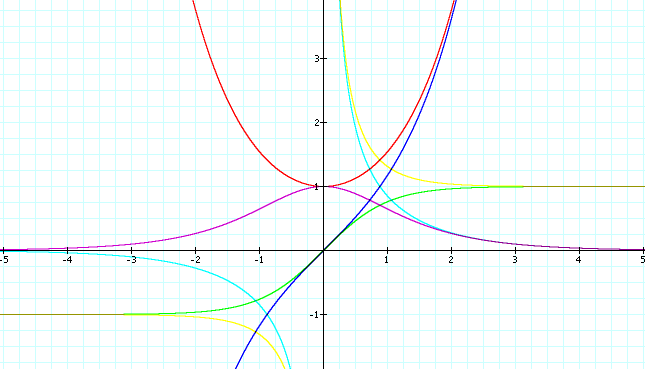Hyperbolic Trig Functions (in the works)The Hyperbolic Trig functions. Sinh (blue), Cosh (red), Tanh (green), Sech (sky-blue), Csch (pink), Coth (yellow).

Description

Sinh is called Hyperbolic Sine. Sinh and 5 other functions are analogous to the trig functions. They are defined this way:

Sinh[x]:= ( E^2-E^(-x) )/2 or -I*Sin[I*x]
Cosh[x]:= ( E^2+E^(-x) )/2 or Cos[I*x]
Tanh[x]:= Sinh[x]/Cosh[x]  or -I*Tan[I*x]

Sech[x]:= 1/Cosh[x]
Csch[x]:= 1/Sinh[x]
Coth[x]:= Cosh[x]/Sinh[x]

The E is the number Limit[(1 + 1/x)^x, x -> ∞] ~= 2.71828. The capital I denotes the complex number Sqrt[-1].

They are called hyperbolic trig functions because they bear strong similarities to the trig functions. Trig functions relate to a circle, while hyperbolic trig functions relate to a rectangular hyperbola x^-y^==1 in a similar way. And all identies of trig functions have a analogue in the hyperbolic ones of the same form.

The hyperbolic cosine Cosh is famously known as catenary .

Related Web Sites

Robert Yates: Curves and Their Properties .